#### Vectors in three Dimensions, Physics tutorial

Vectors in Space:

Magnitude of a Vector in Space:

The axes of reference are defined by the right-hand-rule. Ox, OY and OZ form a right-handed set of rotation from OX to OY takes a right handed corkscrew action along the positive direction of OZ.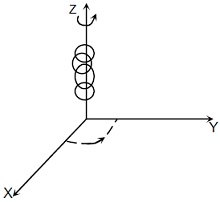Resolution of Vectors in the three mutually perpendicular axes: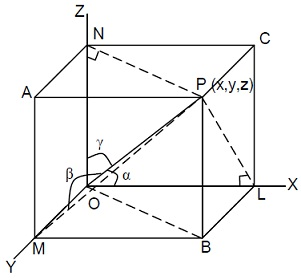Here we wish to determine the vector in space into components in three dimensional rectangular coordinate system. Let the vector OP be situated in a 3-dimensional rectangular coordinate system with its starting point O at the origin shown in Figure.

Let OX, OY and OZ represent the axes. Let the coordinates of OP be (X, Y, Z).

Then, draw the projections of OP and OX, OY and OZ and let these be represented by OL, OM and ON respectively.

If and r are the angles of inclination of OPwith OX, OY and OZ axes respectively,

then,

OP Cos A= x

OP Cos B= y

And

OP Cos r = z

OP2 (Cos2  + Cos2  + Cos2 r) = x2 + y2 + 22

But we know that

OP2 = OL2 + OM2 + ON2 = x2 + y2 + 22

Cos2  + Cos2 + Cos2 r = l2 + m2 + n2 = 1 3.15

where l, m, n are called the direction cosines.

Also op= x2/OP +y2/OP+z2/ OP=(x/OP).x + (y/OP).y+(z/OP).z

Cos αx +cos βy+ cosrz

Lx+my+nz

Therefore vector OP can be complete resolved in magnitude by coordinate of starting point (O, O, O) and end point (X, Y, Z) and in direction by three direction cosines (l, m, n).

Now considering the case when the vector lies in a plane, say the XOY plane, then Z = 0 and we get that OP = lx + my it follows also that for the vector lying in XOZ plane, then y = 0 and for vector lying in YOZ plane, x = 0.

Resolution of Vectors in Three Mutually perpendicular axes in terms of the Unit Vectors:

Just as in two dimensions, we can also indicate three-dimensional vectors is in terms of standard unit vectors, i, j, and k. These vectors are unit vectors in the positive x, y, and z direction, respectively. In terms of coordinates, we can write them as i=(1,0,0), j=(0,1,0), and k=(0,0,1). We can state any three-dimensional vector as the sum of scalar multiples of these unit vectors in form a=(a1,a2,a3)=a1i+a2j+a3k.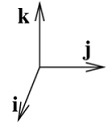Standard unit vectors in three dimensions:

What is the length of vector a= (a1, a2, a3)? We can decompose vector in (a1,a2,a3)=(a1,a2,0)+(0,0,a3), where two vectors on the right hand side stand for two green line segments in above applet. These two line segments form the right triangle whose hypotenuse is vector a. The first vector can be thought of as a two dimensional vector, so its length is ||(a1,a2,0)||= ||(a1,a2)||= √a21+a22. The second vector's length is ||(0,0,a3)||=|a3|. Therefore, by the Pythagorean Theorem, the length of a is

||a||= √||(a1,a2,0)||2+||(0,0,a3)||2= √a21+a22+a23

Vector Product:

Scalar (or Dot) Product:

Multiplication of vectors is same thing as saying product of vectors.

There are two types of products of vectors.

(1) The Scalar Product

(2) The Vector Product

The Scalar Product: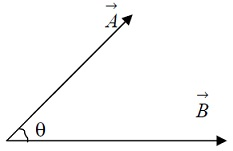The dot product (also called the inner product or scalar product) of two vectors is defined as:

A.B= |A||B|.cosΘ

Where |A| and |B| represents the magnitudes of vectors A and B and theta is the angle between vectors A and B.

Dot product calculation:

The dot or scalar product of vectors vector A a1i+a2j and vector B =b1i+b2jcan be written as:

A.B= a1.b1+a2.b2

Properties of dot Product:

i) a. b is a scalar

ii) a b= ba. i.e. the dot product is commutative

iii) a.(b.c)= a.b+ a.ci.e. the dot product is associative over addition

iv) (ma).b= m(a.b) a.(mb)

v) If a.b=0 and a and bare not zero, vectors then, a is perpendicular to b

vi) |a|= √a2= √a.a

vii) a.a>0 For any non zero vector

viii) a.a = 0 only if a = 0

The Vector (or Cross) Product: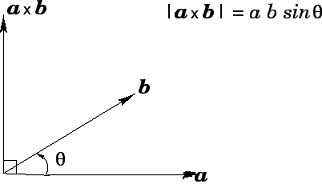In the figure a and b are two vectors. They can be multiplied using the Cross Product. Cross Product a × b of two vectors is another vector which is at right angles to both. We can compute Cross Product this way:

a × b = |a| |b| sin(θ) n

• |a| is the magnitude (length) of vector a
• |b| is the magnitude (length) of vector b
• θ is the angle between a and b
• n is the unit vector at right angles to both a and b

So the length is: length of a times the length of b times the sine of angle between a and b, then we multiply by vector n to ensure it heads in right direction (at right angles to both a and b).

Properties of the vector product are:

i) Ax Bis a vector

ii) A x B= -Bx A

iii) If A and Bare non-zero vectors, and A x B= 0 then Ais parallel to B

iv) A x A = 0, for any vector A

v) (A+ B) x C = (A x C) + (B x C)

That is, the vector product is distributive over addition. Notice that the order in which these vectors appear remains the same.

6. (m A) x B = M (A x B)= A x (mB)

Tutorsglobe: A way to secure high grade in your curriculum (Online Tutoring)

Expand your confidence, grow study skills and improve your grades.

Since 2009, Tutorsglobe has proactively helped millions of students to get better grades in school, college or university and score well in competitive tests with live, one-on-one online tutoring.

Using an advanced developed tutoring system providing little or no wait time, the students are connected on-demand with a tutor at www.tutorsglobe.com. Students work one-on-one, in real-time with a tutor, communicating and studying using a virtual whiteboard technology.  Scientific and mathematical notation, symbols, geometric figures, graphing and freehand drawing can be rendered quickly and easily in the advanced whiteboard.

Free to know our price and packages for online physics tutoring. Chat with us or submit request at info@tutorsglobe.com